# Forestry: Using Trigonmetry to Determine the Hieght of a Tree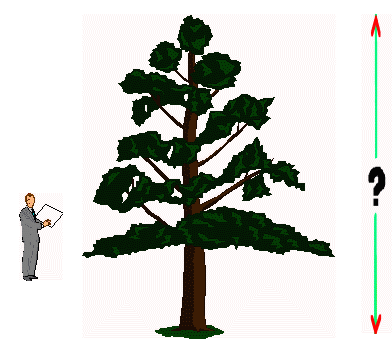I need to know but I don’t want to climb it to find out! There must be an easier way.

Suppose we walk back a measured distance from the tree. We can determine this distance using a tape measure. Then we can sight the top and the bottom of the tree and find the angle each makes with the horizontal. Foresters measure these angles with a device called a clinometer.

### Setting up the problem - translating from words to symbols:

Let’s do this and see what we get. Suppose we walk away from the tree a distance of 35 meters. We then sight the bottom of the tree and find it is 20° below eye level (As we walked back from the tree, we were climbing a hill). A similar sighting on the top of the tree reveals it is 43° above eye level.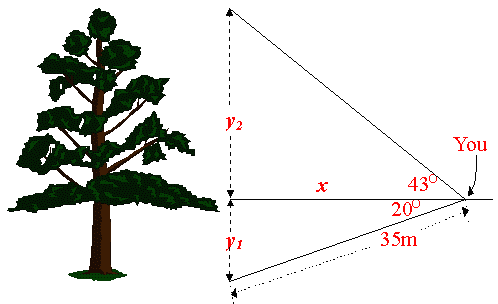Look at the above diagram. The total height of the tree is y1 + y2.

The horizontal distance from you to the tree after you have moved back 35 m is x. Using trigonometric formulas,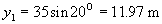y2 can be found in two steps.

1. First find x: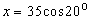2. Then find y2: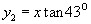By substituting the first equation into the second,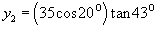or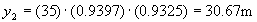The total height is y1 + y2 = 11.97 + 30.67 = 42.64 mWritten by G. John Smith, September 16, 1997